# 1.1 线性模型¶

## 1.1.1 普通最小二乘法

LinearRegression 是拟合一个带有回归系数的， 使得数据的实际观测值和线性近似预测的预测值之间的残差平方和最小的一个线性模型。数学上讲，它解决了这个形式的问题:

LinearRegression将采用它的 fit 方法去拟合数组，并将线性模型的回归系数存储在它的coef_中：

>>> from sklearn import linear_model>>> reg = linear_model.LinearRegression()>>> reg.fit([[0, 0], [1, 1], [2, 2]], [0, 1, 2])LinearRegression()>>> reg.coef_array([0.5, 0.5])

## 1.1.2 岭回归与分类

### 1.1.2.1 回归

Ridge 通过对系数的大小施加惩罚来解决普通最小二乘的一些问题。岭系数最小化一个带惩罚项的残差平方和：

>>> from sklearn import linear_model>>> reg = linear_model.Ridge(alpha=.5)>>> reg.fit([[0, 0], [0, 0], [1, 1]], [0, .1, 1])Ridge(alpha=0.5)>>> reg.coef_array([0.34545455, 0.34545455])>>> reg.intercept_0.13636...

### 1.1.2.2 分类

Ridge 有一个分类器变体：RidgeClassifier。该分类器首先将二分类目标转换为{-1，1}，然后将问题作为一个回归任务处理， 以优化上述目标。预测类对应于回归预测的符号。对于多分类，该问题被视为多输出回归，预测的类对应于具有最高值的输出。

RidgeClassifier可以明显快于LogisticRegression，因为它只计算投影矩阵一次。

### 1.1.2.4 设置正则化参数：广义交叉验证

RidgeCV 通过内置的alpha 参数的控制交叉验证来实现岭回归。 该对象与 GridSearchCV 的使用方法相同，只是它默认为 Generalized Cross-Validation(广义交叉验证 GCV)，这是一种有效的留一交叉验证方法（LOO-CV）:

>>> import numpy as np>>> from sklearn import linear_model>>> reg = linear_model.RidgeCV(alphas=np.logspace(-6, 6, 13))>>> reg.fit([[0, 0], [0, 0], [1, 1]], [0, .1, 1])RidgeCV(alphas=array([1.e-06, 1.e-05, 1.e-04, 1.e-03, 1.e-02, 1.e-01, 1.e+00, 1.e+01,      1.e+02, 1.e+03, 1.e+04, 1.e+05, 1.e+06]))>>> reg.alpha_0.01

“Notes on Regularized Least Squares”, Rifkin & Lippert (technical report, course slides).

## 1.1.3. Lasso

Lasso是一个估计稀疏系数的线性模型。它在某些情况下是有用的，因为它倾向于给出非零系数较少的解，从而有效地减少了给定解所依赖的特征数。 因此，Lasso 及其变体是压缩感知领域的基础。在一定条件下，可以恢复非零系数的精确集合。见压缩感知：用L1优先层重建(Lasso))

Lasso类中的实现采用坐标下降法作为拟合系数的算法。另一个算法 见最小角回归

>>> from sklearn import linear_model>>> reg = linear_model.Lasso(alpha=0.1)>>> reg.fit([[0, 0], [1, 1]], [0, 1])Lasso(alpha=0.1)>>> reg.predict([[1, 1]])array([0.8])

• “Regularization Path For Generalized linear Models by Coordinate Descent”, Friedman, Hastie & Tibshirani, J Stat Softw, 2010 (Paper).
• “An Interior-Point Method for Large-Scale L1-Regularized Least Squares,” S. J. Kim, K. Koh, M. Lustig, S. Boyd and D. Gorinevsky, in IEEE Journal of Selected Topics in Signal Processing, 2007 (Paper)

### 1.1.3.1 设置正则化参数

alpha 参数控制估计系数的稀疏程度。

#### 1.1.3.1.1 使用交叉验证

scikit-learn 设置Lasso alpha参数是通过两个公开的对象，LassoCVLassoLarsCV。其中， LassoLarsCV 是基于下面将要提到的 最小角回归 算法。

#### 1.1.3.1.3 与支持向量机正则化参数的比较

alpha 和 SVM 的正则化参数C 之间的等式关系是 alpha = 1 / C 或者 alpha = 1 / (n_samples * C)，并依赖于估计器和模型优化的确切的目标函数。

## 1.1.4 多任务Lasso

MultiTaskLasso 是一个估计多元回归问题的稀疏系数的线性模型， y是一个二维数组， 形状是(n_samples, n_tasks)。约束条件是，对于所有回归问题（也叫任务），所选的特征是相同的。

## 1.1.5 弹性网络

ElasticNet是一个训练时同时用ℓ1和ℓ2范数进行正则化的线性回归模型。

ElasticNetCV 类可以通过交叉验证来设置参数 alpha） 和 l1_ratio （) 。

• “Regularization Path For Generalized linear Models by Coordinate Descent”, Friedman, Hastie & Tibshirani, J Stat Softw, 2010 (Paper).
• “An Interior-Point Method for Large-Scale L1-Regularized Least Squares,” S. J. Kim, K. Koh, M. Lustig, S. Boyd and D. Gorinevsky, in IEEE Journal of Selected Topics in Signal Processing, 2007 (Paper)

## 1.1.6 多任务弹性网

MultiTaskElasticNet 是一个估计多元回归问题的稀疏系数的弹性网： Y 是一个二维数组， 形状是 (n_samples, n_tasks)。约束条件是，对于所有回归问题（也叫任务），所选的特征是相同的。

MultiTaskElasticNetCV 中可以通过交叉验证来设置参数 alpha） 和 l1_ratio （） 。

## 1.1.7 最小角回归

LARS的优势在于：

• 在特征数明显大于样本数的情况下，它在数值算法上是非常有效的。
• 它的计算速度和前向选择一样快，其复杂度与普通最小二乘法相同。
• 它产生一个完整的分段线性解决方案路径，这在交叉验证或类似的尝试调优模型中很有用。
• 如果两个特征与目标之间的相关性几乎一致，那么它们的系数应以大致相同的速率增加。因此，该算法的性能与我们直觉所期望的一样，而且更稳定。
• 它很容易被修改，以便为其他估计器(如Lasso)提供解。

LARS的缺点包括：

• 因为LARS是基于对残差的迭代修改， 它可能会对噪音的影响特别敏感。这个问题，在 2004 年统计年鉴的文章由 Weisberg 详细讨论过。

LARS模型可以使用估计器LARs，或者其低级实现LARS_PATHLARS_PATH_gram

## 1.1.8 LARS Lasso

LassoLars 是利用LARS算法实现的LASSO模型，与基于坐标下降的LASSO模型不同，它得到的是分段线性的精确解，是其自身系数范数的函数。

>>> from sklearn import linear_model>>> reg = linear_model.LassoLars(alpha=.1)>>> reg.fit([[0, 0], [1, 1]], [0, 1])LassoLars(alpha=0.1)>>> reg.coef_array([0.717157..., 0.        ])

LARS 算法提供了一个几乎无代价的沿着正则化参数的系数的完整路径，因此通常的做法用一个函数 lars_pathlars_path_gram来检索路径。

### 1.1.8.1 数学公式

LARS解不是给出向量结果，而是由一条曲线组成，它表示参数向量的ℓ1范数的每个值的解。完整的系数路径存储在数组coef_path_中，该数组的形状是(n_features, max_features+1)。第一列总是零。

## 1.1.9 正交匹配追踪(OMP)

OrthogonalMatchingPursuitorthogonal_mp近似的拟合了一个线性模型， 该模型对非0系数的数值进行了约束。（比如. ℓ0伪范数)。

OMP的每一步的都是基于贪心算法，在每一步元素都包含与当前残差高度相关的元素。它类似于简单的匹配寻踪(MP)方法，但更好的是，在每次迭代时，在先前选择的字典元素的空间上用正交投影重新计算残差。

## 1.1.10 贝叶斯回归

• 它可以调整以适应手头的数据
• 它可以用于在估计过程中引入正则项。

• 模型的推断可能很费时间。

### 1.1.10.1 贝叶斯岭回归

BayesianRidge估计了上述回归问题的概率模型。系数 的先验由球面高斯给出：

α 和 λ 上的先验是一般服从 gamma分布，这是高斯精度的共轭先验。得到的模型称为贝叶斯岭回归，类似于传统的 Ridge

>>> from sklearn import linear_model>>> X = [[0., 0.], [1., 1.], [2., 2.], [3., 3.]]>>> Y = [0., 1., 2., 3.]>>> reg = linear_model.BayesianRidge()>>> reg.fit(X, Y)BayesianRidge()

>>> reg.predict([[1, 0.]])array([0.50000013])

>>> reg.coef_array([0.49999993, 0.49999993])

Section 3.3 in Christopher M. Bishop: Pattern Recognition and Machine Learning, 2006
David J. C. MacKay, Bayesian Interpolation, 1992.
Michael E. Tipping, Sparse Bayesian Learning and the Relevance Vector Machine, 2001.

### 1.1.10.2 自动关联判定-ARD

ARDRegressionBayesian Ridge Regression,非常相似，但会导致系数   更加稀疏。ARD回归在系数上具有不同的先验性，因为它放弃了高斯球面的假设。

Bayesian Ridge Regression相比，的每个坐标都有各自的标准差。而的先验被选择为是给定超参数的相同的gamma分布。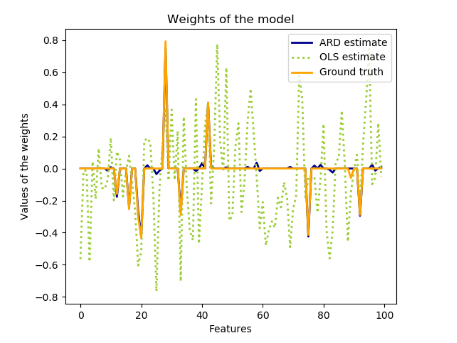ARD在文献中也被称为稀疏贝叶斯学习和相关向量机。 

1Christopher M. Bishop: Pattern Recognition and Machine Learning, Chapter 7.2.1

2David Wipf and Srikantan Nagarajan: A new view of automatic relevance determination

3Michael E. Tipping: Sparse Bayesian Learning and the Relevance Vector Machine

4Tristan Fletcher: Relevance Vector Machines explained

## 1.1.11 Logistic回归

Logistic回归，尽管它的名称有"回归"，但是却是一个线性的分类模型。Logistic回归在文献中也被称为logit回归、最大熵分类(MaxEnt)或对数线性分类器。在这个模型中， 描述单个实验输出结果的可能性概率用的logistic function

Logistic回归的实现是在LogisticRegression， 在这里实现了二分类(binary), 一对多分类(One-vs-Rest，一对剩下), 和多项式Logistic回归， 并且可以选择的正则化有ℓ1、ℓ2或弹性网。

LogisticRegression类中实现的求解方法包括： “liblinear”, “newton-cg”, “lbfgs”, “sag” and “saga”。

“sag”优化方法用的是随机平均梯度下降( Stochastic Average Gradient descent) 6， 当样本数和特征数都很大时，它比其他的优化方法更快。

“saga”是 “sag”的一种变体， 它同样支持非光滑(non-smooth)的ℓ1正则化( penalty="l1"), 因此对于稀疏型的logistic回归这是一个不错的选择， 它也是唯一一个支持弹性网正则化(penalty="elasticnet")的优化方案。

“lbfgs”是一种类似于Broyden-Fletcher-Goldfarb-Shanno算法的优化方法， 是一种属于拟牛顿(quasi-Newton)的方法。“lbfgs”建议用于小数据集， 对于大型数据集表现会受到影响。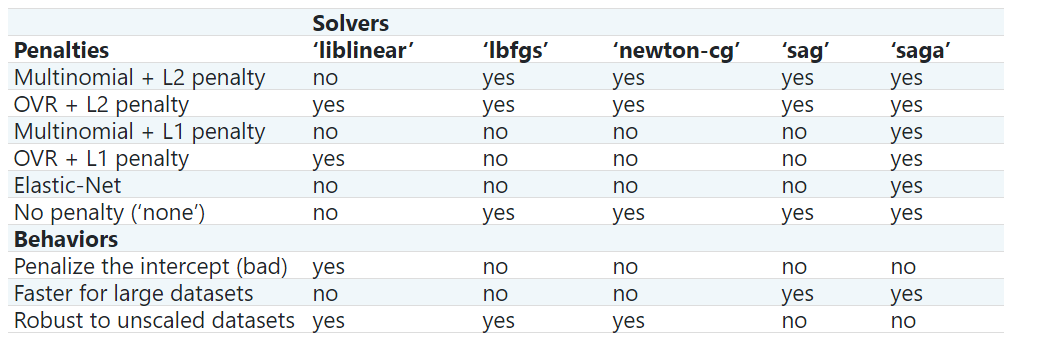因为“lbfgs”鲁棒性更强， 所以默认是“lbfgs”。对于大型数据集，“saga”解决程序通常更快。对于大型数据集，您还可以考虑使用对数损失( ‘log’ loss)的 SGDClassifier，这可能会更快，但需要更多的调优。

fit_intercept=False, 并且拟合得到的coef_或者待预测的数据为 0 时， solver=liblinearLogisticRegression或者LinearSVC与直接调用外部的liblinear library相比， 在得分上可能有些差异。这是因为对于决策函数是0的样本， LogisticRegressionLinearSVC预测为负类(negative class), 而 liblinear 库则会预测为正类。注意， 一个fit_intercept=False并且很多样本的决策函数为0的模型，很有可能是一个欠拟合 、糟糕的模型， 你应该设置fit_intercept=True 并且增大intercept_scaling

LogisticRegressionCV实现了内置交叉验证支持下的Logistic回归，根据得分属性找到最优的Cl1_ratio。 “newton-cg”, “sag”, “saga” 和 “lbfgs”被发现对于高维的密集数据会更快， 原因是热启动(warm-starting)(见术语表)。

5Christopher M. Bishop: Pattern Recognition and Machine Learning, Chapter 4.3.4

6Mark Schmidt, Nicolas Le Roux, and Francis Bach: Minimizing Finite Sums with the Stochastic Average Gradient.

7Aaron Defazio, Francis Bach, Simon Lacoste-Julien: SAGA: A Fast Incremental Gradient Method With Support for Non-Strongly Convex Composite Objectives.

## 1.1.12 广义线性回归（Generalized Linear Regression）

α是L2正则化惩罚项。提供样本权重后，平均值即为加权平均值。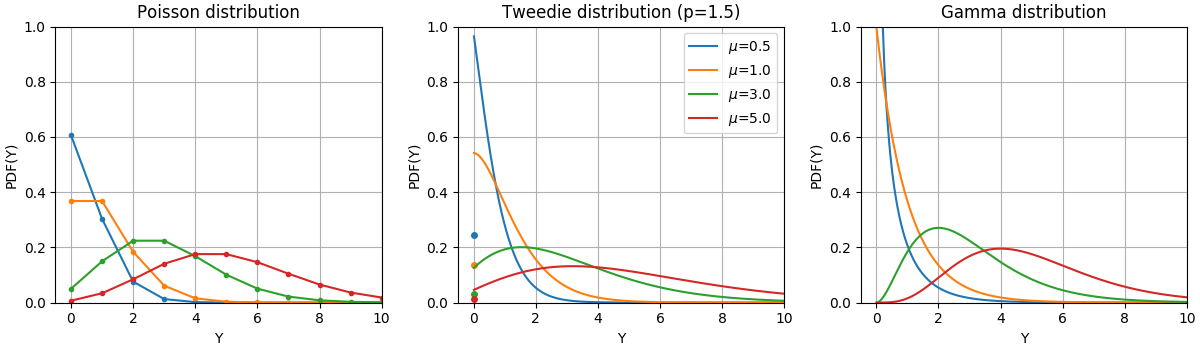根据泊松分布、Tweedie分布(幂=1.5)和具有不同平均值的伽马分布()的随机变量 的PDF。观察泊松分布和Tweedie(幂=1.5)分布在 的点质量，但不观察严格为正的目标域的伽马分布。

• 如果目标值似乎比伽马分布的尾部更重，那么您可以尝试使用逆高斯偏差(或者更高的Tweedie族方差)。

• 农业/天气建模:每年降雨的次数(Poisson)，每次的降雨量(Gamma)，每年总降雨量(Tweedie /复合Poisson Gamma)。

• 风险建模/保单定价:每年索赔事件/投保人的数量(Poisson)，每个索赔事件的成本(Gamma)，每个投保人每年的总成本(Tweedie /复合Poisson Gamma)。

• 预测维护:每年生产中断事件的数量:Poisson，中断持续时间:Gamma，每年总中断时间(Tweedie /复式Poisson Gamma)。

• 10McCullagh, Peter; Nelder, John (1989). Generalized Linear Models, Second Edition. Boca Raton: Chapman and Hall/CRC. ISBN 0-412-31760-5.
• 11Jørgensen, B. (1992). The theory of exponential dispersion models and analysis of deviance. Monografias de matemática, no. 51. See also Exponential dispersion model.

### 1.1.12.1. 使用

TweedieRegressor为Tweedie分布实现了一个广义线性模型，该模型允许使用适当的功率参数对上述任何分布进行建模。特别是:

• power = 0: 正态分布。在这种情况下，诸如 Ridge, ElasticNet 等特定的估计器通常更合适。

• power = 1: 泊松分布。方便起见可以使用 PoissonRegressor 。然而，它完全等同于 TweedieRegressor(power=1, link='log').

• power = 2: 伽马分布。方便起见可以使用GammaRegressor 。然而，它完全等同于 TweedieRegressor(power=2, link='log').

• power = 3: 逆高斯分布。

>>> from sklearn.linear_model import TweedieRegressor>>> reg = TweedieRegressor(power=1, alpha=0.5, link='log')>>> reg.fit([[0, 0], [0, 1], [2, 2]], [0, 1, 2])TweedieRegressor(alpha=0.5, link='log', power=1)>>> reg.coef_array([0.2463..., 0.4337...])>>> reg.intercept_-0.7638...

Examples:

## 1.1.13 随机梯度下降（SGD）

SGDClassifierSGDRegressor类提供了使用不同的(凸)损失函数和不同的正则项， 去拟合一个线性的回归模型或者分类模型。比如， 设定loss="log", SGDClassifier 会拟合一个Logistic回归模型。而如果设定loss="hinge"则会拟合一个线性支持向量机 (SVM)。

## 1.1.14 感知机(Perceptron)

Perceptron 是另一种适用于大规模学习的简单分类算法。有如下默认：

• 它不需要设置学习率
• 它不需要正则项
• 它只用错误样本更新模型

## 1.1.15 被动感知算法(Passive Aggressive Algorithms)

“Online Passive-Aggressive Algorithms” K. Crammer, O. Dekel, J. Keshat, S. Shalev-Shwartz, Y. Singer - JMLR 7 (2006)

## 1.1.16 鲁棒回归(Robustness regression)：离群(outliers)与模型错误

### 1.1.16.1 不同的场景和有用的概念

• 离群值到底是在还是在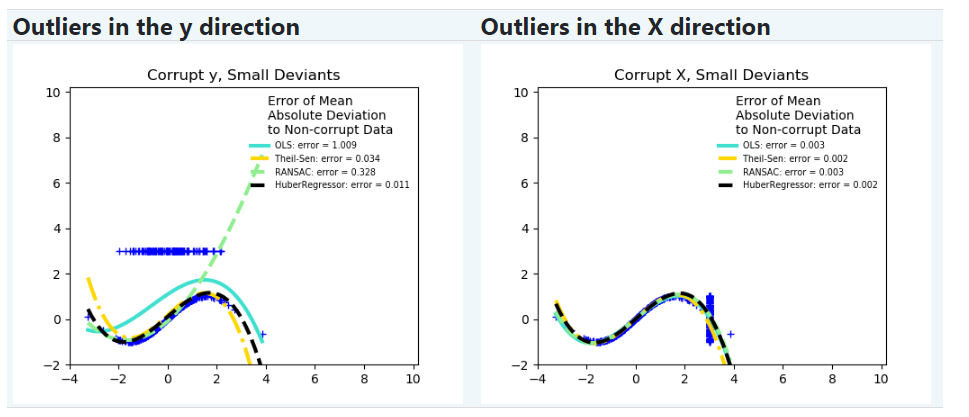​ 离群值在方向上 离群值在方向上

• 离群值的比例VS误差的幅度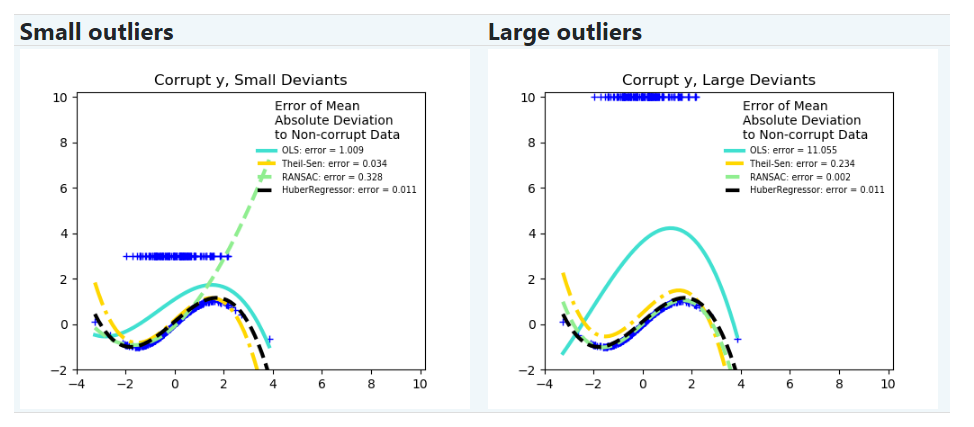​ 小离群 大离群

Scikit-learn提供了3种鲁棒回归的估计器：RANSAC, Theil SenHuberRegressor
HuberRegressor 一般快于 RANSACTheil Sen ，除非样本数很大，即 n_samples >> n_features 。 这是因为 RANSACTheil Sen 都是基于数据的较小子集进行拟合。但使用默认参数时， Theil SenRANSAC 可能不如 HuberRegressor 鲁棒。
RANSACTheil Sen快很多， 并且这种快的程度会随着样本数量的增加而加深。
RANSAC将更好地处理方向的大型离群值(最常见的情况)。
Theil Sen将更好地处理方向的中型离群值，但该能力将在高维设置中消失。

### 1.1.16.2 RANSAC：随机抽样一致性(RANdom SAmple Consensus)

RANSAC (RANdom SAmple Consensus)能够使用完整数据集中的样本点组成的随机子集拟合模型。

RANSAC是一种不确定的算法，它以一定概率产生一个合理的结果，而这还取决于迭代次数(见参数 max_trials)它通常用于线性和非线性回归问题，在计算机视觉领域尤其流行。

#### 1.1.16.2.1 算法细节

1. 从原始数据中随机选择min_samples个样本，并检查数据集是否有效(请参阅is_data_valid)
2. 将模型拟合到随机子集上(base_estimator.fit)，并检查估计的模型是否有效(请参阅is_model_valid)
3. 通过计算模型的残差(base_estimator.predict(X) - y), 如果样本绝对残差小于residual_threshold就会被认为是局内点。按照这种方式将数据分为局内点和离群点。
4. 当内部的局内样本数达到最大时，模型达到最优就保存下来。如果当前的估计模型有相同的局内点，只有当它有更好的分数时，它才被认为是最好的模型。

### 1.1.16.3 Theil-Sen估计：基于广义中值的估计(generalized-median-based estimator)

TheilSenRegressor估计器使用高纬度下的中位数的一个泛化性。因此，它对多元离群值具有很强的鲁棒性。但是，请注意，随着维数的增加，估计量的鲁棒性迅速下降。在高维数据上失去鲁棒性， 就不会比普通最小二乘法好。

https://en.wikipedia.org/wiki/Theil%E2%80%93Sen_estimator

#### 1.1.16.3.1 理论细节

TheilSenRegressor与(普通最小二乘）Ordinary Least Squares (OLS)在渐进效率和无偏估计方面相当。与普通最小二乘相比， Theil-Sen是一种非参数的方法， 这意味着它对数据的基本分布没有任何假定。由于Theil-Sen是一种基于中值的估计器，因此它对损坏的数据(也称为离群值)具有更强的鲁棒性。在简单线性回归的情况下，Theil-Sen的崩溃点(breakdown point)为29.3%，这意味着它可以容忍高达29.3%的损坏数据。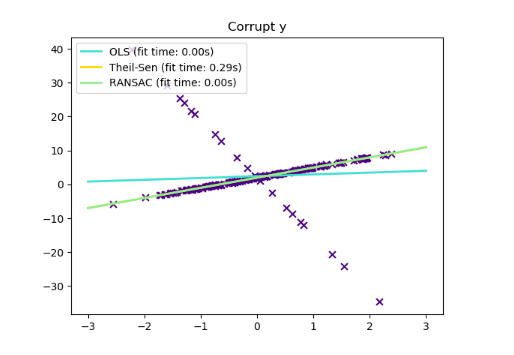TheilSenRegressor在scikit-learn中的实现遵循了对多元线性回归模型的推广，该模型使用空间中值，它是多维中值的推广。

10Xin Dang, Hanxiang Peng, Xueqin Wang and Heping Zhang: Theil-Sen Estimators in a Multiple Linear Regression Model.

1120.Kärkkäinen and S. Äyrämö: On Computation of Spatial Median for Robust Data Mining.

### 1.1.16.4 Huber回归

HuberRegressorRidge不同， 因为它把线性损失应用于被分为离群值的样本。如果样本的绝对误差小于某一阈值，则该样本被归类为局内样本(inlier)。它也不同于 TheilSenRegressorRANSACRegressor，因为它没有忽略离群值的影响，而是给予它们较小的权重。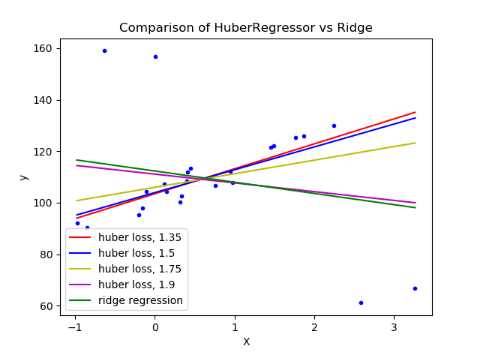给出了HuberRegressor要最小化的损失函数：

### 1.1.16.5 注意

Peter J. Huber, Elvezio M. Ronchetti: Robust Statistics, Concomitant scale estimates, pg 172

## 1.1.17 多项式回归：用基函数扩展线性模型

(有时令人惊讶的)观察到，这仍然是一个线性模型：要看到这一点，想象一下创建一组新的特征。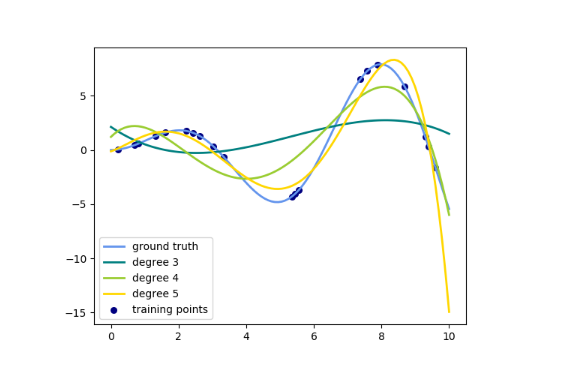此图是使用 PolynomialFeatures转换器创建的，该转换器将输入数据矩阵转换为给定度数的新数据矩阵。使用如下：

>>> from sklearn.preprocessing import PolynomialFeatures>>> import numpy as np>>> X = np.arange(6).reshape(3, 2)>>> Xarray([[0, 1],       [2, 3],       [4, 5]])>>> poly = PolynomialFeatures(degree=2)>>> poly.fit_transform(X)array([[ 1.,  0.,  1.,  0.,  0.,  1.],       [ 1.,  2.,  3.,  4.,  6.,  9.],       [ 1.,  4.,  5., 16., 20., 25.]])

>>> from sklearn.preprocessing import PolynomialFeatures>>> from sklearn.linear_model import LinearRegression>>> from sklearn.pipeline import Pipeline>>> import numpy as np>>> model = Pipeline([('poly', PolynomialFeatures(degree=3)),...                   ('linear', LinearRegression(fit_intercept=False))])>>> # fit to an order-3 polynomial data>>> x = np.arange(5)>>> y = 3 - 2 * x + x ** 2 - x ** 3>>> model = model.fit(x[:, np.newaxis], y)>>> model.named_steps['linear'].coef_array([ 3., -2.,  1., -1.])

>>> from sklearn.linear_model import Perceptron>>> from sklearn.preprocessing import PolynomialFeatures>>> import numpy as np>>> X = np.array([[0, 0], [0, 1], [1, 0], [1, 1]])>>> y = X[:, 0] ^ X[:, 1]>>> yarray([0, 1, 1, 0])>>> X = PolynomialFeatures(interaction_only=True).fit_transform(X).astype(int)>>> Xarray([[1, 0, 0, 0],       [1, 0, 1, 0],       [1, 1, 0, 0],       [1, 1, 1, 1]])>>> clf = Perceptron(fit_intercept=False, max_iter=10, tol=None,...                  shuffle=False).fit(X, y)

>>> clf.predict(X)array([0, 1, 1, 0])>>> clf.score(X, y)1.0